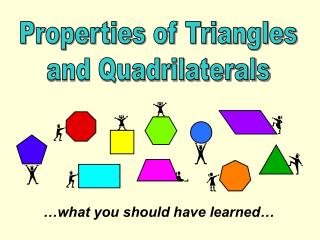Download Presentation…what you should have learned…

# …what you should have learned…

Download Presentation## …what you should have learned…

- - - - - - - - - - - - - - - - - - - - - - - - - - - E N D - - - - - - - - - - - - - - - - - - - - - - - - - - -
##### Presentation Transcript

1. Properties of Triangles and Quadrilaterals …what you should have learned…

2. Investigation #1: Midpoints of Triangle Sides - Length • ‘x’ is half the length of ‘y’ y x • ‘y’ is twice the length of ‘x’ • If ‘x’ is 6 cm long, ‘y’ is….. 12 cm • If ‘y’ is 32 m long, ‘x’ is….. 16 cm

3. y x Investigation #2: Midpoints of Triangle Sides - Slopes  the lines are parallel

4. Diagonals of Parallelograms • the diagonals of parallelograms intersect at their midpoints.

5. Midpoints of Quadrilateral Sides • when the midpoints of any quadrilateral are connected, the interior figure is always a parallelogram.

6. Verifying Properties of Geometric Figures What does it mean to ‘verify’? Prove Give evidence

7. To verify… • Draw a labeled diagram • Decide what properties you will need to show are true • Complete calculations • Make ‘therefore statements’ to conclude that the conditions for the specified figure are met.

8. #1 – parallelogram • Opposite sides are parallel • Opposite sides are equal in length

9. #2 – right triangle • Two sides are perpendicular (have negative reciprocal slopes)

10. #3 – rhombus • Opposite sides are parallel • All sides are equal in length

11. #4 – rectangle • Adjacent sides are perpendicular • Opposite side are equal in length